## Events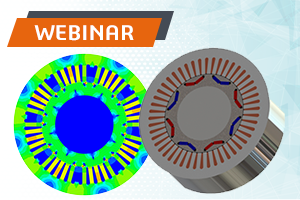## How to Optimize Magnet Size for BLDC Motors in SOLIDWORKS

Tuesday, June 23, 2020
02:00 PM EDT, 06:00 PM GMT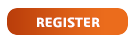## ELECTRO-DYNAMIC-THERMAL SIMULATION OF A VOICE COIL ACTUATOR USING EMS INSIDE SOLIDWORKS

In this article, a voice coil actuator will be studied. A series of simulations, including static force calculation using parametric sweep study, electromechanical analyses and electrothermal simulation, will be performed. EMS, SOLIDWORKS and SOLIDWORKS Motion will be used to carry out the different simulations of the studied voice coil actuator.

Figure 1 shows experimental  and simulation results of the Lorentz force computed at both different DC currents and stroke values. Back emf results versus different speed values are plotted in Figure 2.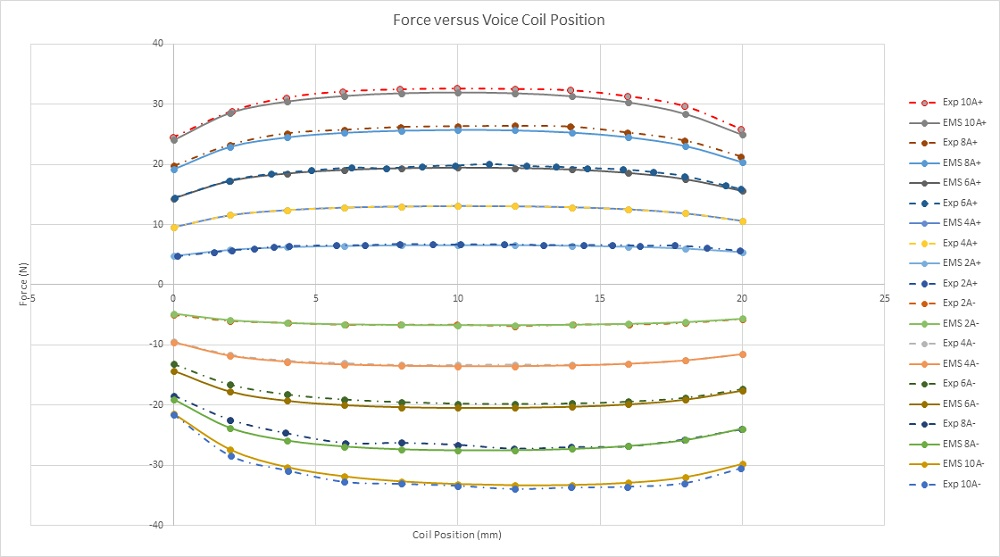Figure 1 - Force results versus coil position and applied current rates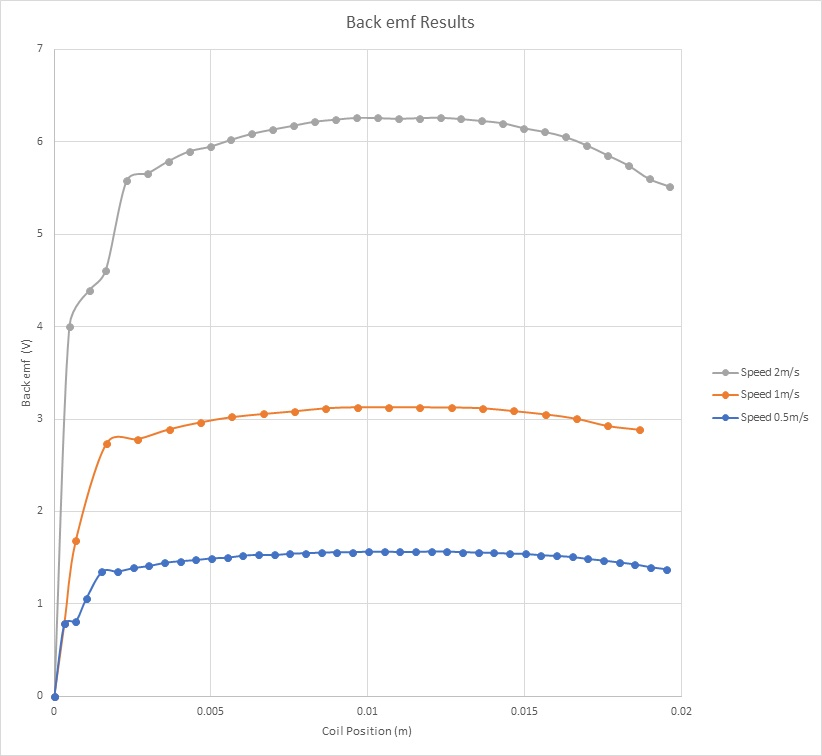Figure 2 - Back emf results

Figure 3 contains several plots of the force results at the same static position (12mm). The plotted curves of the force measured by experimental tests contain few oscillations before achieving the steady state. A force of 4.5N is obtained when a DC voltage of 10V is applied while it is 26N when the applied DC voltage is 60V. The higher the voltage the higher the force is.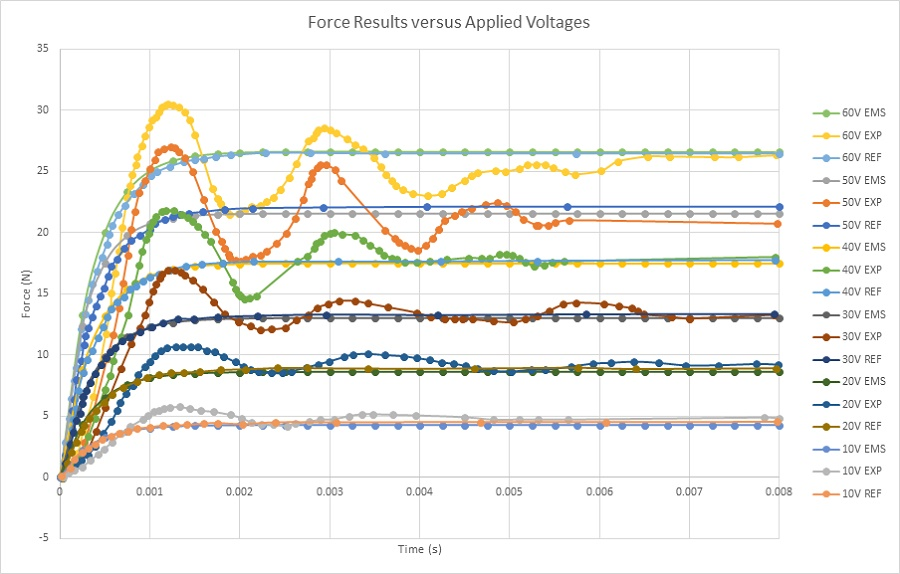Figure 3 - Force results at static position

Electrodynamic simulation is established to calculate and generate electromechanical results including magnetic fields, current, coil position, speed, and acceleration, etc. EMS coupled to SOLIDWORKS motion analysis is used to run these simulations.

Figures 4a) and 4b) display the results of the coil linear displacement and speed for different applied voltages. The maximum speed is reached when the applied voltage is 200V.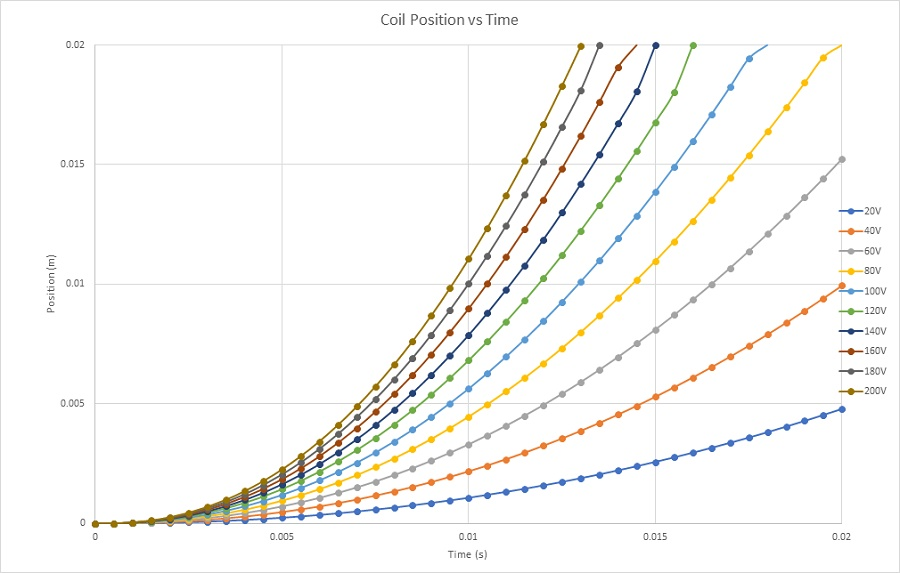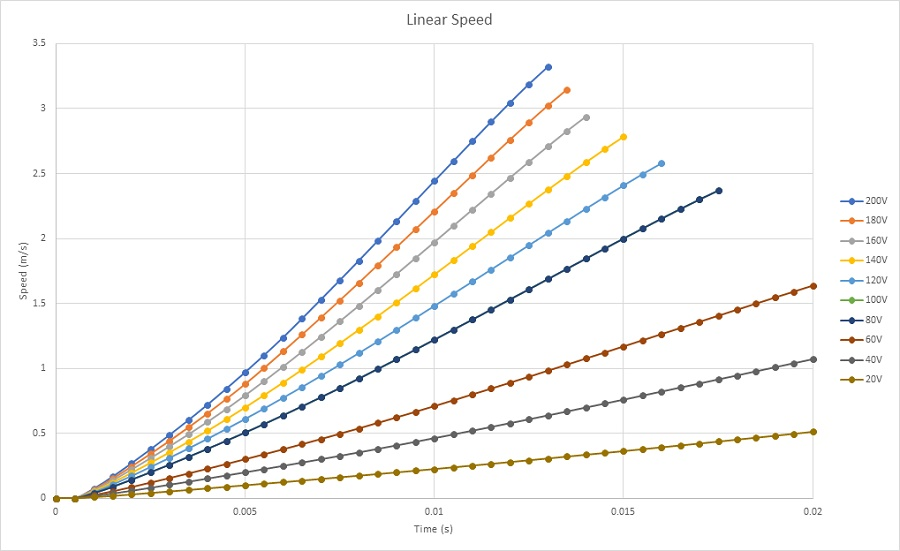Figure 4 - a) Coil displacement, b) Coil speed

Figures 5a) and 5b) display the final temperature of the voice coil actuator for 20V and 200V, respectively. The temperature is generated inside the coil, due to copper loss, then it propagates to the whole system by conduction and convection.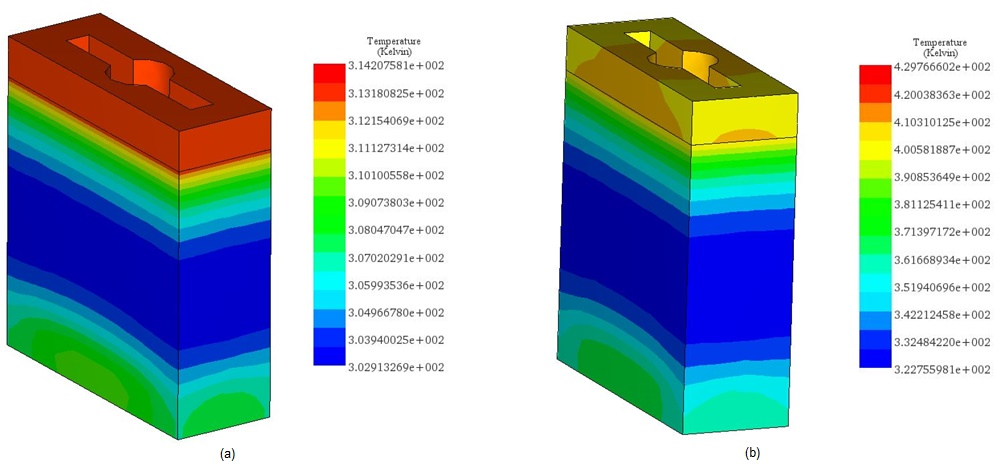Figure 5 - Temperature results, a) 20V, b) 200V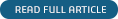## Analysis of a Highly Nonlinear Lorentz Force Linear Motion Electromagnetic Actuator Using EMS

Ian Hunter, Ph.D. and Serge Lafontaine, Ph.D Massachusetts, USA.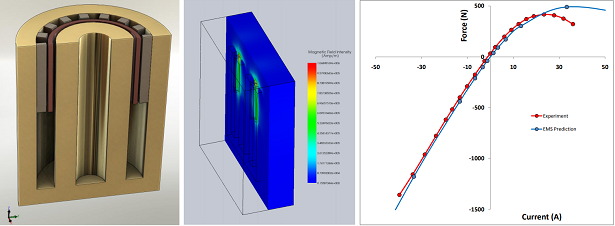More whitepapers